# Exploring the relationships between thaats in Hindustani Music

(24 Nov 2018) music kala

# On thaats: the diff graph

The wikipedia article on Thaats lists the following 10 Hindustani Raags along with the most famous raag associated in the third column.

 1 Bilaval Bilaval S R G M P D N Ś Ionian 29th Dheerashankarabharanam 2 Kafi Kafi S R g M P D n Ś Dorian 22nd Kharaharapriya 3 Bhairavi Bhairavi S r g M P d n Ś Phrygian 8th Hanumatodi 4 Kalyan Yaman S R G m P D N Ś Lydian 65th (Mecha) Kalyani 5 Khamaj Khamaj S R G M P D n Ś Mixolydian 28th Harikambhoji 6 Asavari Asavari S R g M P d n Ś Aeolian 20th Natabhairavi 7 Bhairav Bhairav S r G M P d N Ś Double Harmonic 15th Mayamalavagowla 8 Marva Marva S r G m P D N Ś - 53rd Gamanashrama 9 Poorvi Poorvi S r G m P d N Ś - 51st Kamavardhani 10 Todi Miyan ki Todi S r g m P d N Ś - 45th Shubhapantuvarali

We asked the question, if we only consider the swaras associated with each thaat as a string, can we investigate the graph of thaats constructed with edge-weights as the string diff of the notes.

 num name notes 1 Bilaval RGMPDN 2 Kafi RgMPDn 3 Bhairavi rgMPdn 4 Kalyan RGmPDN 5 Khamaj RGMPDn 6 Asavari RgMPdn 7 Bhairav rGMPdN 8 Marva rGmPDN 9 Poorvi rGmPdN 10 Todi rgmPdN

Let us first construct the Graph. I write the edge list to file first.

``````    import pandas as pd
from networkx import *
import matplotlib.pyplot as plt

DF=pd.DataFrame(data)
DF.columns=DF.iloc
DF = DF.drop(0)

def cmp(A, B):
count = 0
for a,b in zip(A,B):
if a != b:
count += 1
return count

with open('thaat_edge_list.txt', 'w') as f:
for i in range(1,11):
for j in range(i+1,11):
E = cmp(str(list(DF['notes'].loc[DF['num'] == i])), str(list(DF['notes'].loc[DF['num'] == j])))
f.write(str(i) + '\t'+ str(j) + '\t' + str(E) + '\n')

nodetype=int,
encoding='utf-8')
``````

We can now visualize the graph by coloring the edges by the edge weight.

``````    colordict = {1:'g',
2:'w',
3:'w',#'orange',#'orange',
4:'w',#'r',
5:'w'}#'k'}

nodes = [i for i in range(1,11)]
labels = {i:DF['name'][i] for i in range(1,11)}
draw_circular(G,with_labels=True,nodelist=nodes,
edge_color=[colordict[G[i][j]['weight']] for i,j in G.edges()],
node_color='w',
labels=labels)
plt.savefig('thaat_diff-1.png',dpi=300)
``````

The green edges show edge weight of 1, i.e. these thaats differ by one note.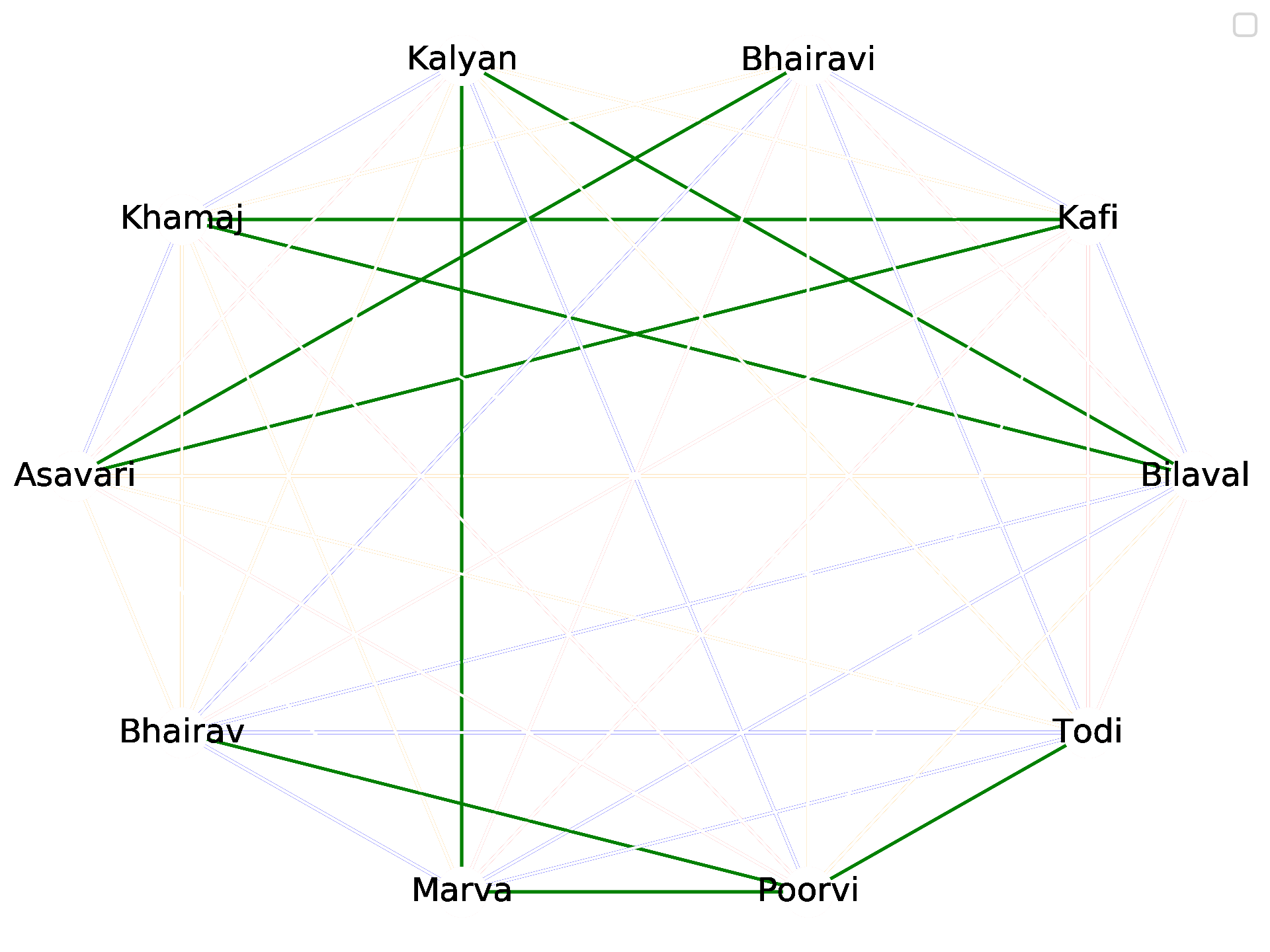``````    colordict = {1:'g',
2:'b',
3:'w',#'orange',#'orange',
4:'w',#'r',
5:'w'}#'k'}

nodes = [i for i in range(1,11)]
labels = {i:DF['name'][i] for i in range(1,11)}
draw_circular(G,with_labels=True,nodelist=nodes,
#width=[G[i][j]['weight'] for i,j in G.edges()]
edge_color=[colordict[G[i][j]['weight']] for i,j in G.edges()],
node_color='w',
labels=labels)
plt.savefig('thaat_diff-1,2.png',dpi=300)
``````

These include thaats that differ by one or two notes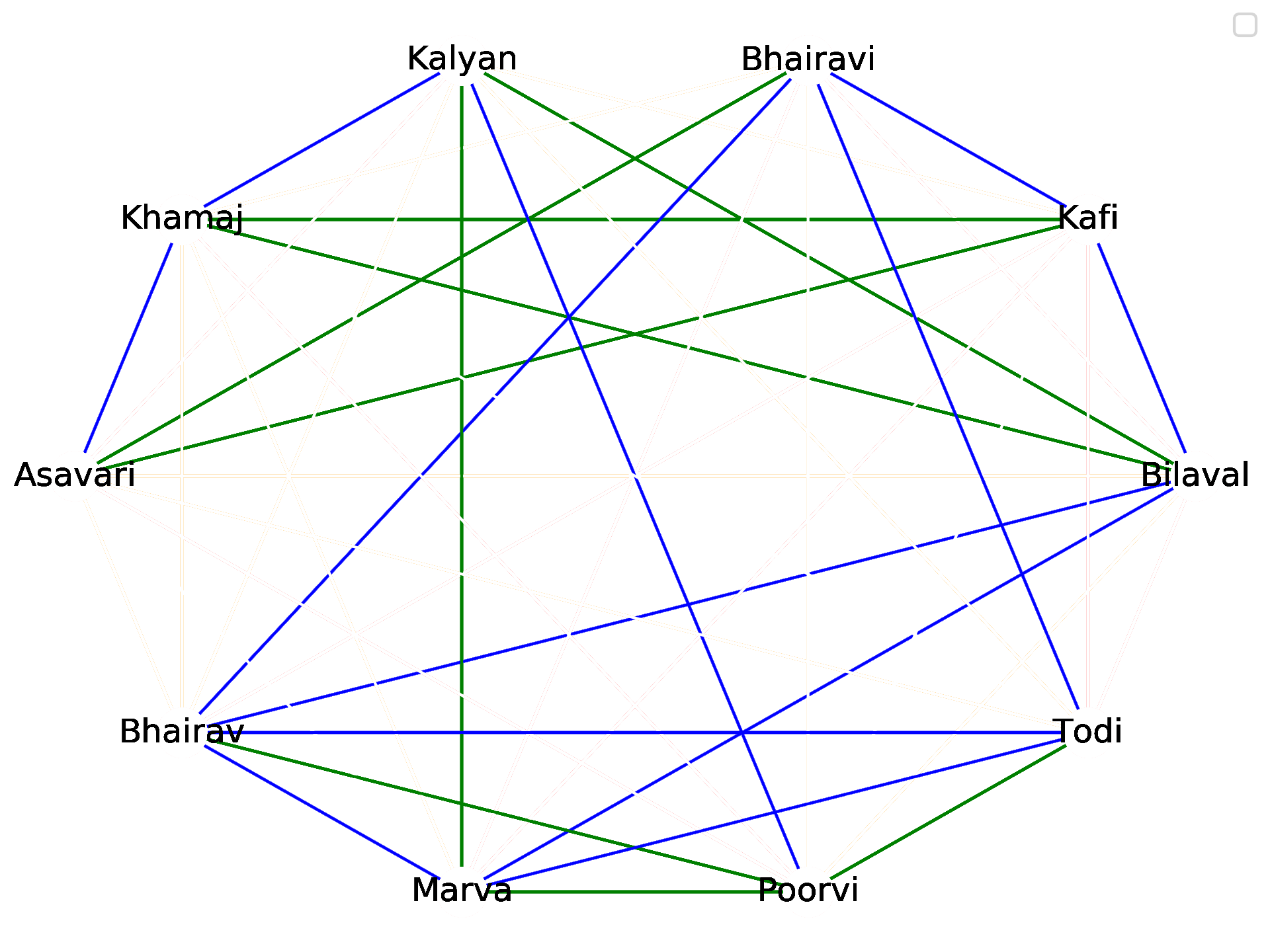``````    colordict = {1:'g',
2:'b',
3:'orange',#'orange',
4:'w',#'r',
5:'w'}#'k'}

nodes = [i for i in range(1,11)]
labels = {i:DF['name'][i] for i in range(1,11)}
draw_circular(G,with_labels=True,nodelist=nodes,
#width=[G[i][j]['weight'] for i,j in G.edges()]
edge_color=[colordict[G[i][j]['weight']] for i,j in G.edges()],
node_color='w',
labels=labels)
plt.savefig('thaat_diff-1,2,3.png',dpi=300)
``````

.. and those that differ by three…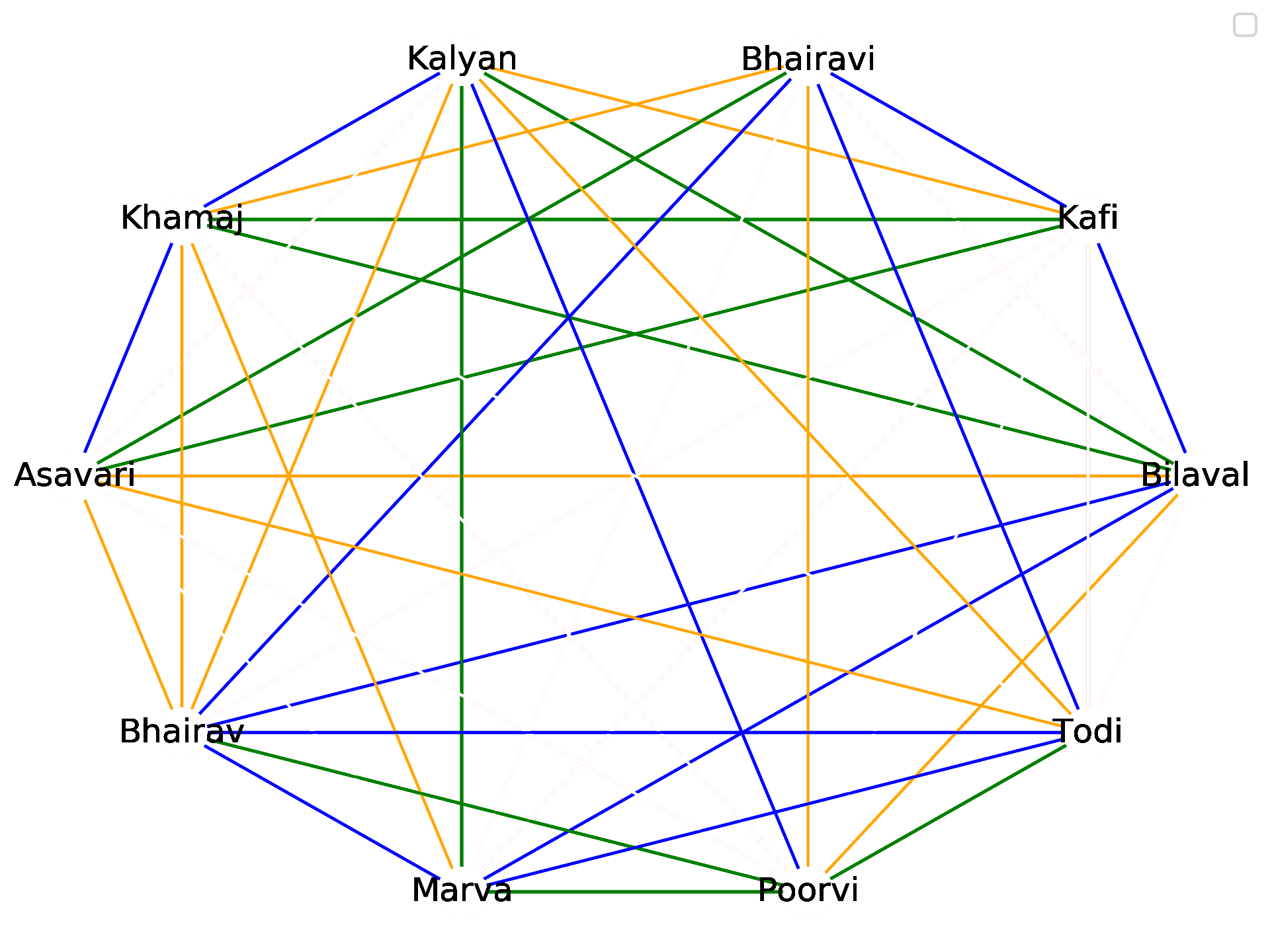``````    colordict = {1:'g',
2:'b',
3:'orange',#'orange',
4:'r',
5:'w'}#'k'}

nodes = [i for i in range(1,11)]
labels = {i:DF['name'][i] for i in range(1,11)}
draw_circular(G,with_labels=True,nodelist=nodes,
#width=[G[i][j]['weight'] for i,j in G.edges()]
edge_color=[colordict[G[i][j]['weight']] for i,j in G.edges()],
node_color='w',
labels=labels)
plt.savefig('thaat_diff-1,2,3,4.png',dpi=300)
``````

… and finally up to four notes (in red).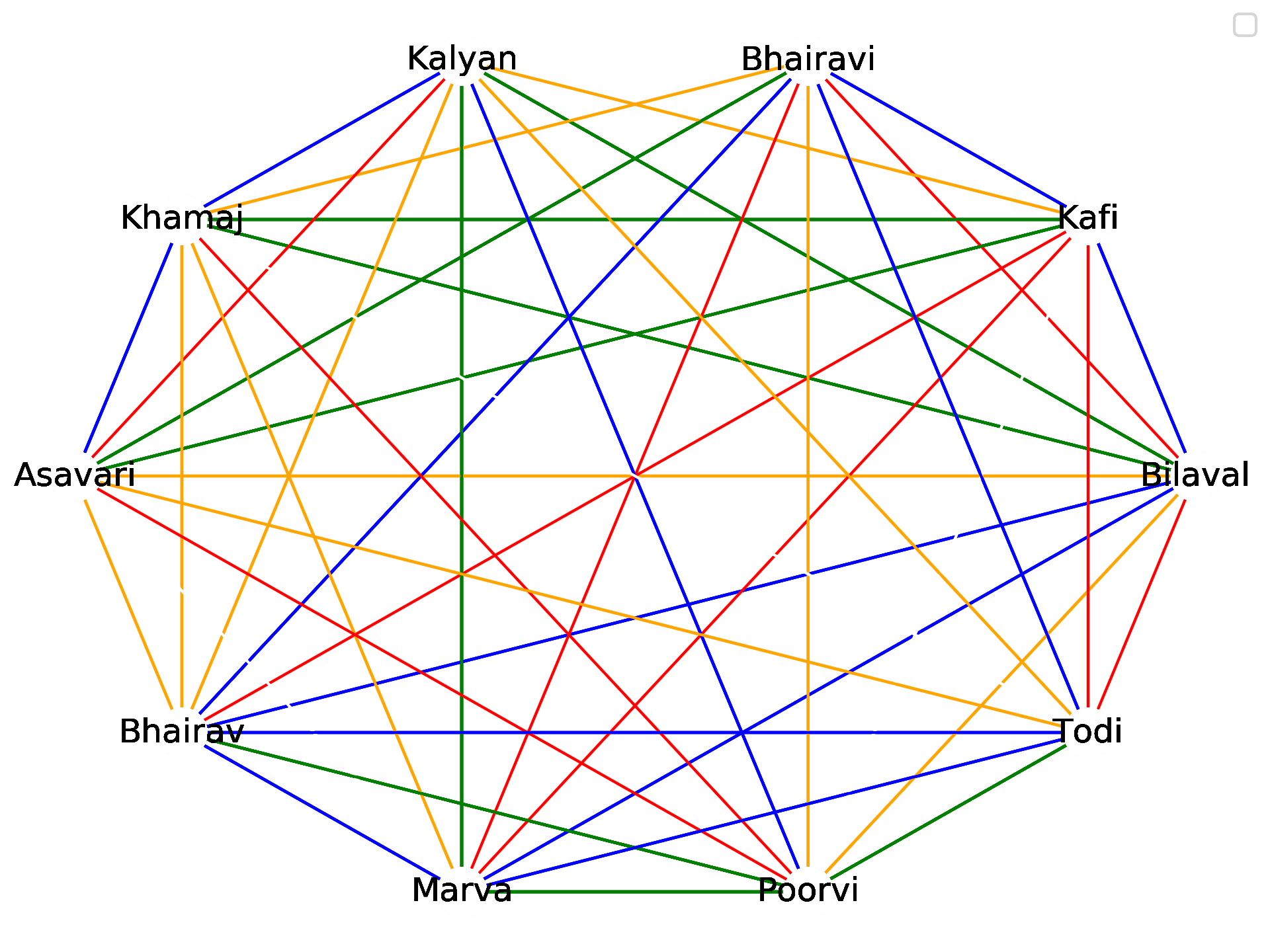What thaats are similar to all other thaats? Look at number of thaats at most 2 notes different

``````    degreedict = {}
for i in range(1,11):
count =0
degreedict[i] = {'count':0,'name':labels[i]}
for j in range(1, 11):
if  i!=j and G[i][j]['weight'] <= 2.0:
count += 1
degreedict[i]['count'] = count

for k in degreedict.keys():
print(degreedict[k]['name'],degreedict[k]['count'])
``````
``````('Bilaval', 5)
('Kafi', 4)
('Bhairavi', 4)
('Kalyan', 4)
('Khamaj', 4)
('Asavari', 3)
('Bhairav', 5)
('Marva', 5)
('Poorvi', 4)
('Todi', 4)
``````

What thaats are most different from other thaats? Look at number of thaats that are more than 3 notes different.

``````    degreedict = {}
for i in range(1,11):
count =0
degreedict[i] = {'count':0,'name':labels[i]}
for j in range(1, 11):
if  i!=j and G[i][j]['weight'] > 3.0:
count += 1
degreedict[i]['count'] = count

for k in degreedict.keys():
print(degreedict[k]['name'],degreedict[k]['count'])
``````
``````('Bilaval', 2)
('Kafi', 4)
('Bhairavi', 3)
('Kalyan', 2)
('Khamaj', 2)
('Asavari', 3)
('Bhairav', 1)
('Marva', 3)
('Poorvi', 3)
('Todi', 3)
``````

Putting it all together as an animation makes it clear that for the most part thaats are equally different from each other.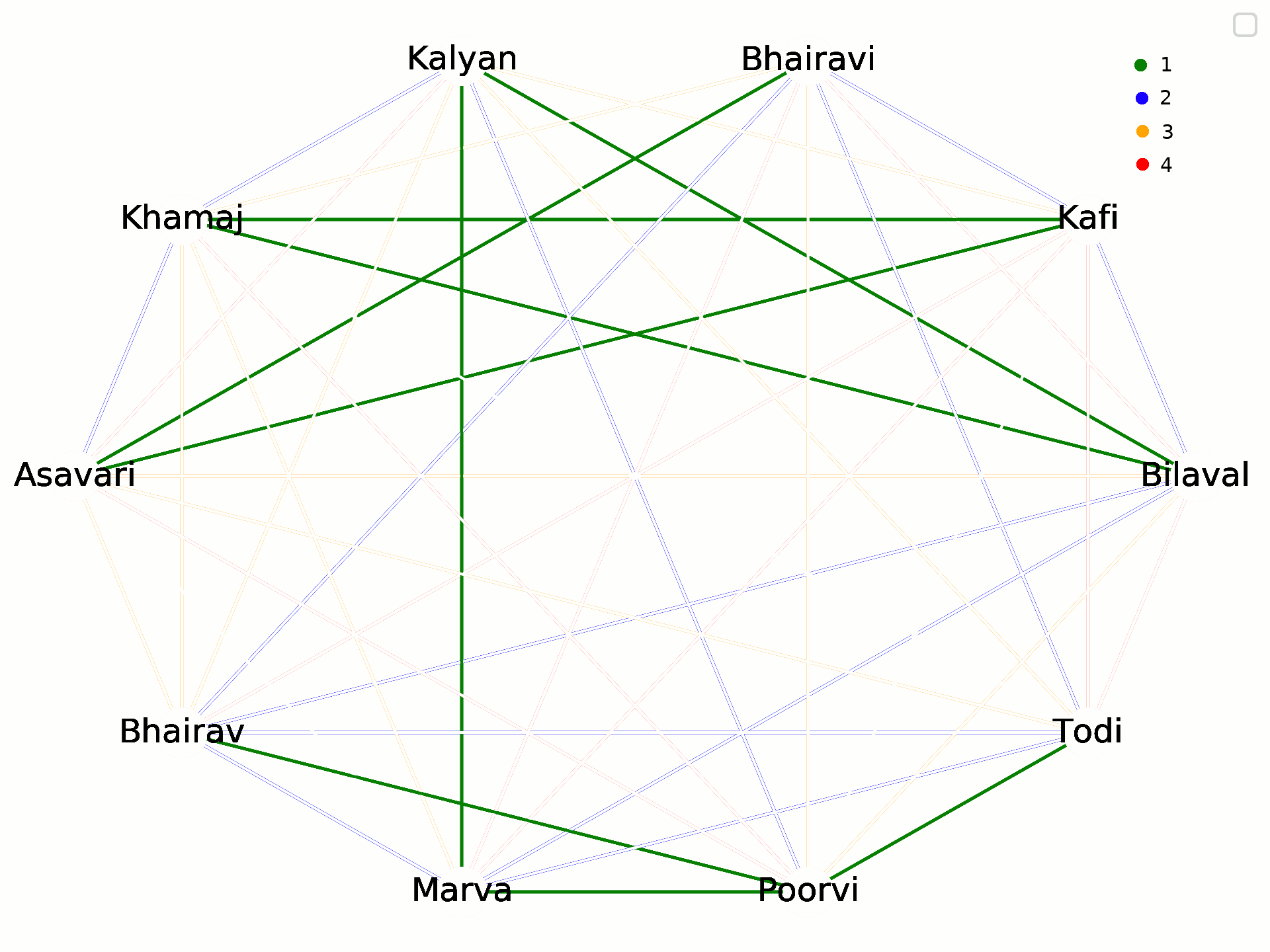While these are purely abstract relationships between thaats, we will be exploring the significance of these relationships on the raags that constitute each thaat next week!